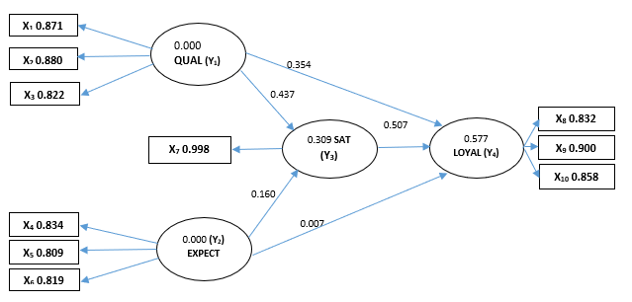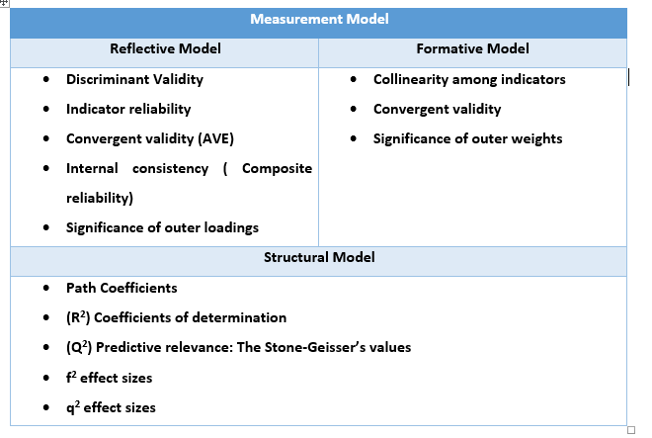# Cross-eyed PhD: Tip 12 SEM Models (Part 3)

posted in: Wealth of learning | 0
###### Piled High and Deep”, www.PHDCOMICS.com, by Jorge Cham

Editor’s Note: Concluding post on Structural Equation Modeling by contributing author, Sanjay Malla, Research Scholar develops the discussion on SEM with an  example exploring a patron satisfaction model vs data. I really like the way he brings out the implications for the model studies in future – the fact is that in research we hypothesize a model and (sometimes) get carried away by the theory. However, in research, theory has to follow the data- that is how and when you will earn your PhD!

## Beginners guide to Structure Equation Modeling (SEM) Part – III

### Evaluating PLS-SEM results

In our previous article on SEM, we focused on outer model (also known as Measurement model) and explained how the latent variables (constructs) are measured in two different ways; formative or reflective. In this series, we shall be evaluating PLS-SEM model with both the formative and reflective measurements using a dummy survey data on patron satisfaction.

Patron satisfaction (Y3) is a multidimensional latent construct that is difficult to measure directly. It can be measured indirectly with a set of measurable items, also known as indicators. In our survey example we have identified four latent constructs, namely Quality (Y1), Expectation (Y2), Satisfaction (Y3) and Loyalty (Y4). These four constructs are measured using specially designed questions on a Likert scale and the final framework is shown below using a path model diagram.

##### Figure 1: PLS-SEM ResultsThe PLS path modeling estimation for measuring patron satisfaction shown in Figure 1 has 3 important numbers which help us explain the overall model:

1. R2 values of the latent endogenous construct (Numbers in the circles)
2. Path Coefficients for the structural model relationship ( Numbers on the arrows)

Model estimation gives us empirical measures of the relationships between the items and the constructs as well as between the constructs. It basically enables us to compare the theoretical model with reality as projected by the final set of sample data. So it’s about understanding the fitness of theory and data. For our assessment of PLS-SEM model, some basic elements necessary to explain the model must be covered in the research as shown in Exhibit 1. The process involves separate assessment of measurement models (Reflective & Formative) and the structural model.

##### Exhibit 1Using the PLS-SEM path diagram in Figure 1. We make following observations:

##### Explanation of the Dependent Endogenous variable variance –
• The coefficient of determination, R2, is 0.577 for the (Y4) LOYAL endogenous construct. Which means that the other three latent constructs (QUAL, EXPECT and SAT) moderately explain 57.7% of the variance in LOYAL construct.

R2  possible values range from 0 to 1. In research, R2 of 0.75 is substantial, 0.50 is moderate, and 0.25 is weak)

• QUAL (Y1) and EXPECT (Y2) together explain 30.9% of the variance in SAT (Y3).

(SAT (Y3) acts both as independent and dependent construct in the model)

##### Explanation of Inner Path model-
• We observe that SAT (Y3) 0.507 has the strongest impact on LOYAL (Y4), followed by QUAL (Y1) 0.354 and EXPECT (Y2) 0.007.
• The path relationship between QUAL (Y1) and LOYAL (Y4) is statistically significant.
• The path relationship between SAT (Y3) and LOYAL (Y4) is also statistically significant.
• However, the path relationship between EXPECT (Y2) and LOYAL (Y4) is not statistically significant. This is mainly because its path coefficient (0.007) is lower than 0.1, therefore we can sum up by explaining: constructs SAT and QUAL are both moderately strong predictors of construct LOYAL, but construct EXPECT does not directly predict endogenous LOYAL.
##### Other parameters that researcher should interpret from PLS-SEM reports-
• Internal Consistency reliability: composite reliability should be 0.7 or higher. If it is an exploratory research, 0.6 or higher is acceptable ( Bagozzi and Yi, 1998)
• Indicator reliability: The indicators our loadings should be 0.70 or higher. In case of exploratory research , 0.4 or higher is acceptable (Hulland, 1999)
• Convergent Validity: Measured in terms of AVE numbers, it should be 0.5 or higher (Bagozzi and Yi, 1988)
• Discriminant Validity: (Fornell and Larcker, 1981) suggest that the “square root” of AVE of each latent variable should be greater than the correlations among the other latent variables.
• Outer Weights: They are the relationships in formative model and determine an item’s relative contribution to its assigned construct.
• Outer Loadings: These are estimated relationships in reflective models. They determine an item’s absolute contribution to its assigned construct.
• Collinearity of indicators: Each indicator’s tolerance (VIF) value should be higher that 0.20 (lower than 5).
• Critical t value: It’s the cut off on which the significance of a coefficient is determined. Typical critical t values are 2.57, 1.96 and 1.65 for a significance level of 1%, 5% and 10% respectively (two-tailed tests)
• F2 effect size: It is a measure used to access the relative impact of a predictors construct on an endogenous construct.

(Effect size of 0.02, 0.15 and 0.35 indicate small, medium and large effects)

• Q2 effect size: It is a measure to access the relative predictive relevance of a predictor construct on an endogenous construct.

(Effect size of 0.02, 0.15 and 0.35 indicate small, medium and large effects)

Let’s now look at the PLS-SEM results in totality and interpret it for business leaders and key decision makers by understanding the relationships among various latent constructs: perceived quality (QUAL) Y1, patron expectations (EXPECT) Y2, patron satisfaction (SAT) Y3 and patron loyalty (LOYAL) Y4.  Through the survey and structured equation modeling using ADANCO 2.1.1, it’s observed that patrons care about food quality X1* (0.871), Services X2* (0.880) and billing efficiency X3* (0.822) respectively. (X1, 2, 3 are indicators & numbers within brackets are their loadings). As all the loading are above 0.8, they are all good indicators of perceived quality construct (QUAL, Y1). Quality is an important construct here as it significantly impacts patron satisfaction levels which will determine their intentions to revisit the place, and give positive recommendations about the place.

Looking at patron expectations (EXPECT, Y2) construct, it’s observed that food variety X4 (0.834), ambience X5 (0.809) and well-groomed staff X6 (0.819) are all good indicators of patron expectations with all loadings above 0.8. From the path diagram, while these patron expectations can make patrons satisfied, any correction in these expectation levels does not necessarily make them loyal due to the weak path relationship (0.007) between (EXPECT), Y2 and (LOYAL), Y4. In terms of resource allocations, decision makers must focus on improving (QUAL), Y1 as it is a moderately strong predictor of construct (LOYAL).

Further analysis of the inner model in the path diagram shows that constructs (QUAL), Y1 and (EXPECT), Y2 together only explain 30.9% variance in (SAT), Y3 construct. This finding indicates that while carrying out future research, decision makers must think of other factors when assessing satisfaction levels and patron loyalty.

Acknowledgement: I would like to express my sincere gratitude to all my mentors who have patiently guided me on the subject and encouraged me to explore more about this robust and advance technique. Thank you all!

Sanjay Malla

Doctoral Scholar

Semiconductor & Embedded Systems Professional